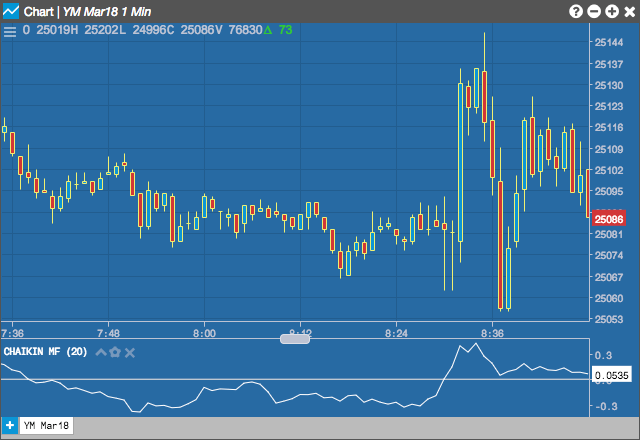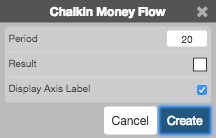Charts

# Chaikin Money Flow (CMF)

The Chaikin Money Flow (CMF) indicator measures Money Flow Volume over a period of time to determine buying and selling pressure by comparing the closing price in relation to the high-low range and the volume. When the CMF is above zero, it can indicate buying pressure; likewise, it indicates selling pressure when the CMF is below zero. Values farther away from zero indicate greater pressures.

When CMF crosses the zero Line, it can identify a potential trend reversal.## Configuration Options• Period: Number of bars to use in the calculations.
• Color Selectors: Colors to use for graph elements.
• Display Axis Label: Whether to display the most recent value on the Y axis.

## Formula

$CMF = \frac{\sum_{1}^{n} Money\;Flow\;Volume}{\sum_{1}^{n} Volume}$

where:

$Money\;Flow\;Volume = Money\;Flow\;Multiplier_{n} \times Volume_{n}$

$Money\;Flow\;Multipler = \frac{\left ( (Close_{n}-Low_{n}) - (High_{n} - Close_{n}) \right )}{(High_{n}-Low_{n})}$